MODEL Statement

MODEL response = <effects> </ options> ;

MODEL events/trials = <effects> </ options> ;

The MODEL statement specifies the response, or dependent variable, and the effects, or explanatory variables. If you omit the explanatory variables, the procedure fits an intercept-only model. An intercept term is included in the model by default. The intercept can be removed with the NOINT option.

You can specify the response in the form of a single variable or in the form of a ratio of two variables denoted events/trials. The first form is applicable to all responses. The second form is applicable only to summarized binomial response data. When each observation in the input data set contains the number of events (for example, successes) and the number of trials from a set of binomial trials, use the events/trials syntax.

In the events/trials model syntax, you specify two variables that contain the event and trial counts. These two variables are separated by a slash (/). The values of both events and (trials–events) must be nonnegative, and the value of the trials variable must be greater than 0 for an observation to be valid. The variable events or trials can take noninteger values.

When each observation in the input data set contains a single trial from a binomial or multinomial experiment, use the first form of the preceding MODEL statements. The response variable can be numeric or character. The ordering of response levels is critical in these models. You can use the RORDER= option in the PROC GENMOD statement to specify the response level ordering.

Responses for the Poisson distribution must be all nonnegative, but they can be noninteger values.

The effects in the MODEL statement consist of an explanatory variable or combination of variables. Explanatory variables can be continuous or classification variables. Classification variables can be character or numeric. Explanatory variables representing nominal, or classification, data must be declared in a CLASS statement. Interactions between variables can also be included as effects. Columns of the design matrix are automatically generated for classification variables and interactions. The syntax for specification of effects is the same as for the GLM procedure. See the section Specification of Effects for more information. Also see Chapter 42: The GLM Procedure.

Table 40.7 summarizes the options available in the MODEL statement.

Table 40.7: MODEL Statement Options

Option

Description

Specifies the subpopulations

Sets the confidence coefficient

Sets the convergence criterion for profile likelihood confidence intervals

Displays confidence limits for predicted values

Uses effect coding for all classification variables

Sets the convergence criterion

Sets the relative Hessian convergence criterion

Displays the parameter estimate correlation matrix

Displays the parameter estimate covariance matrix

Displays case deletion diagnostic statistics

Specifies the built-in probability distribution

Names a variable used for performing an exact Poisson regression

Computes covariances and associated statistics using the expected Fisher information matrix

Displays the values of variable in the input data set in the OBSTATS table

Sets initial values for parameter estimates

Initializes the intercept term

Displays the iteration history for all iterative processes

Computes the maximum likelihood estimate and confidence limits of k basedComputes two-sided confidence intervals for the partially likelihood function

Sets the maximum allowable number of iterations for all iterative computation processes

Requests that no intercept term

Computes the maximum likelihood estimate and confidence limits of k based on k

Holds the scale parameter fixed

Displays an additional table of statistics

Specifies a variable in the input data set to be used as an offset

Displays predicted values and associated statistics

Displays residuals and standardized residuals

Sets the value used for the scale

Computes the Hessian matrix using the Fisher scoring method

Sets the tolerance for testing singularity

Performs a Type 1 analysis

Computes statistics for Type 3 contrasts

Requests Wald statistics for Type 3 contrasts

Computes two-sided Wald confidence intervals

Includes the regression variables in the OBSTATS table

You can specify the following options in the MODEL statement after a slash (/).

AGGREGATE= (variable-list)
AGGREGATE= variable
AGGREGATE

specifies the subpopulations on which the Pearson chi-square and the deviance are calculated. This option applies only to the multinomial distribution or the binomial distribution with binary (single trial syntax) response. It is ignored if specified for other cases. Observations with common values in the given list of variables are regarded as coming from the same subpopulation. This affects the computation of the deviance and Pearson chi-square statistics. Variables in the list can be any variables in the input data set. Specifying the AGGREGATE option is equivalent to specifying the AGGREGATE= option with a variable list that includes all explanatory variables in the MODEL statement. Pearson chi-square and deviance statistics are not computed for multinomial models unless this option is specified.

ALPHA=number
ALPH=number
A=number

sets the confidence coefficient for parameter confidence intervals to 1–number. The value of number must be between 0 and 1. The default value of number is 0.05.

CICONV=number

sets the convergence criterion for profile likelihood confidence intervals. See the section Confidence Intervals for Parameters for the definition of convergence. The value of number must be between 0 and 1. By default, CICONV=1E–4.

CL

requests that confidence limits for predicted values be displayed (see the OBSTATS option).

CODING=EFFECT
CODING=FULLRANK

specifies that effect coding be used for all classification variables in the model. This is the same as specifying PARAM=EFFECT as a CLASS statement option.

CONVERGE=number

sets the convergence criterion. The value of number must be between 0 and 1. The iterations are considered to have converged when the maximum change in the parameter estimates between iteration steps is less than the value specified. The change is a relative change if the parameter is greater than 0.01 in absolute value; otherwise, it is an absolute change. By default, CONVERGE=1E–4. This convergence criterion is used in parameter estimation for a single model fit, Type 1 statistics, and likelihood ratio statistics for Type 3 analyses and CONTRAST statements.

CONVH=number

sets the relative Hessian convergence criterion. The value of number must be between 0 and 1. After convergence is determined with the change in parameter criterion specified with the CONVERGE= option, the quantity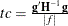is computed and compared to number, where g is the gradient vector, H is the Hessian matrix for the model parameters, and f is the log-likelihood function. If tc is greater than number, a warning that the relative Hessian convergence criterion has been exceeded is printed. This criterion detects the occasional case where the change in parameter convergence criterion is satisfied, but a maximum in the log-likelihood function has not been attained. By default, CONVH=1E–4.

CORRB

requests that the parameter estimate correlation matrix be displayed.

COVB

requests that the parameter estimate covariance matrix be displayed.

DIAGNOSTICS
INFLUENCE

requests that case deletion diagnostic statistics be displayed (see the OBSTATS option).

DIST=keyword
D=keyword
ERROR=keyword
ERR=keyword

specifies the built-in probability distribution to use in the model. If you specify the DIST= option and you omit a user-defined link function, a default link function is chosen as displayed in the following table. If you specify no distribution and no link function, then the GENMOD procedure defaults to the normal distribution with the identity link function. Models for data with correlated responses fit by the GEE method are not available for the zero-inflated distributions.

DIST=

Distribution

BINOMIAL | BIN | B

Binomial

Logit

GAMMA | GAM | G

Gamma

Inverse ( power(–1) )

GEOMETRIC | GEOM

Geometric

Log

IGAUSSIAN | IG

Inverse Gaussian

Inverse squared ( power(–2) )

MULTINOMIAL | MULT

Multinomial

Cumulative logit

NEGBIN | NB

Negative binomial

Log

NORMAL | NOR | N

Normal

Identity

POISSON | POI | P

Poisson

Log

ZIP

Zero-inflated Poisson

Log/logit

ZINB

Zero-inflated negative binomial

Log/logit

EXACTMAX<=variable>

names a variable used for performing an exact Poisson regression. For each observation, the integer part of the EXACTMAX value should be nonnegative and at least as large as the response value. If the EXACTMAX option is specified without a variable, then default values are computed. See the section Exact Logistic and Exact Poisson Regression for information about using this option.

EXPECTED

requests that the expected Fisher information matrix be used to compute parameter estimate covariances and the associated statistics. The default action is to use the observed Fisher information matrix. This option does not affect the model fitting, only the way in which the covariance matrix is computed (see the SCORING= option.)

ID=variable

causes the values of variable in the input data set to be displayed in the OBSTATS table. If an explicit format for variable has been defined, the formatted values are displayed. If the OBSTATS option is not specified, this option has no effect.

INITIAL=numbers

sets initial values for parameter estimates in the model. The default initial parameter values are weighted least squares estimates based on using the response data as the initial mean estimate. This option can be useful in case of convergence difficulty. The intercept parameter is initialized with the INTERCEPT= option and is not included here. The values are assigned to the variables in the MODEL statement in the same order in which they appear in the MODEL statement. The order of levels for CLASS variables is determined by the ORDER= option. Note that some levels of classification variables can be aliased; that is, they correspond to linearly dependent parameters that are not estimated by the procedure. Initial values must be assigned to all levels of classification variables, regardless of whether they are aliased or not. The procedure ignores initial values corresponding to parameters not being estimated. If you specify a BY statement, all classification variables must take on the same number of levels in each BY group. Otherwise, classification variables in some of the BY groups are assigned incorrect initial values. Types of INITIAL= specifications are illustrated in the following table.

Type of List

Specification

List separated by blanks

INITIAL = 3 4 5

List separated by commas

INITIAL = 3, 4, 5

x to y

INITIAL = 3 to 5

x to y by z

INITIAL = 3 to 5 by 1

Combination of list types

INITIAL = 1, 3 to 5, 9

INTERCEPT=number
INTERCEPT=number-list

initializes the intercept term to number for parameter estimation. If you specify both the INTERCEPT= and the NOINT options, the intercept term is not estimated, but an intercept term of number is included in the model. If you specify a multinomial model for ordinal data, you can specify a number-list for the multiple intercepts in the model.

ITPRINT

displays the iteration history for all iterative processes: parameter estimation, fitting constrained models for contrasts and Type 3 analyses, and profile likelihood confidence intervals. The last evaluation of the gradient and the negative of the Hessian (second derivative) matrix are also displayed for parameter estimation. If you perform a Bayesian analysis by specifying the BAYES statement, the iteration history for computing the mode of the posterior distribution is also displayed.

This option might result in a large amount of displayed output, especially if some of the optional iterative processes are selected.

specifies the link function to use in the model. The keywords and their associated built-in link functions are as follows.

CUMCLL

CCLL

Cumulative complementary log-log

CUMLOGIT

CLOGIT

Cumulative logit

CUMPROBIT

CPROBIT

Cumulative probit

CLOGLOG

CLL

Complementary log-log

IDENTITY

ID

Identity

LOG

Log

LOGIT

Logit

PROBIT

Probit

POWER(number) | POW(number)

Power with= number

If no LINK= option is supplied and there is a user-defined link function, the user-defined link function is used. If you specify neither the LINK= option nor a user-defined link function, then the default canonical link function is used if you specify the DIST= option. Otherwise, if you omit the DIST= option, the identity link function is used.

The cumulative link functions are appropriate only for the multinomial distribution.

LOGNB

specifies that the maximum likelihood estimate and confidence limits of the negative binomial dispersion parameter k be computed based. This is the default method used for the negative binomial dispersion parameter, so that specifying no option or specifying the LOGNB option have the same effect. The GENMOD procedure computes the maximum likelihood estimate ofand computes confidence limits based on the asymptotic normality ofrather than of k. The results are always reported in terms of k rather than of. This method ensures that the estimate and confidence limits for k are positive. See Meeker and Escobar (1998, p. 163) for details about this method of computing confidence limits.

LRCI

requests that two-sided confidence intervals for all model parameters be computed based on the profile likelihood function. This is sometimes called the partially maximized likelihood function. See the section Confidence Intervals for Parameters for more information about the profile likelihood function. This computation is iterative and can consume a relatively large amount of CPU time. The confidence coefficient can be selected with the ALPHA=number option. The resulting confidence coefficient is 1–number. The default confidence coefficient is 0.95.

MAXITER=number
MAXIT=number

sets the maximum allowable number of iterations for all iterative computation processes in PROC GENMOD. By default, MAXITER=50.

NOINT

requests that no intercept term be included in the model. An intercept is included unless this option is specified.

NOLOGNB

specifies that the maximum likelihood estimate and confidence limits of the negative binomial dispersion parameter k be computed based on k rather than. If this option is not specified, then the GENMOD procedure computes the maximum likelihood estimate ofand computes confidence limits based on the asymptotic normality ofrather than of k. The results are always reported in terms of k rather than of. This method ensures that the estimate and confidence limits for k are positive. See Meeker and Escobar (1998, p. 163) for details about this method of computing confidence limits.

NOSCALE

holds the scale parameter fixed. Otherwise, for the normal, inverse Gaussian, and gamma distributions, the scale parameter is estimated by maximum likelihood. If you omit the SCALE= option, the scale parameter is fixed at the value 1.

OBSTATS

specifies that an additional table of statistics be displayed. Formulas for the statistics are given in the section Predicted Values of the Mean, the section Residuals, and the section Case Deletion Diagnostic Statistics. Residuals and fit diagnostics are not computed for multinomial models.

For each observation, the following items are displayed:

• the value of the response variable (variables if the data are binomial), frequency, and weight variables

• the values of the regression variables

• predicted mean,, where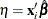is the linear predictor and g is the link function. If there is an offset, it is included in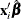.

• estimate of the linear predictor. If there is an offset, it is included in.

• standard error of the linear predictor• the value of the Hessian weight at the final iteration

• lower confidence limit of the predicted value of the mean. The confidence coefficient is specified with the ALPHA= option. See the section Confidence Intervals on Predicted Values for the computational method.

• upper confidence limit of the predicted value of the mean

• raw residual, defined as
• Pearson, or chi residual, defined as the square root of the contribution for the observation to the Pearson chi-square—that is,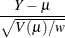where Y is the response,
is the predicted mean, w is the value of the prior weight variable specified in a WEIGHT statement, and V() is the variance function evaluated at.

• the standardized Pearson residual

• deviance residual, defined as the square root of the deviance contribution for the observation, with sign equal to the sign of the raw residual

• the standardized deviance residual

• the likelihood residual

• a Cook distance type statistic for assessing the influence of individual observations on overall model fit

• observation leverage

• DFBETA, defined as an approximation to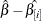for each parameter estimate, whereis the parameter estimate with the ith observation deleted

• standardized DFBETA, defined as DFBETA, normalized by its standard deviation

• zero inflation probability for zero-inflated models

The following additional cluster deletion diagnostic statistics are created and displayed for each cluster if a REPEATED statement is specified:

• a Cook distance type statistic for assessing the influence of entire clusters on overall model fit

• a studentized Cook distance for assessing influence of clusters

• cluster leverage

• cluster DFBETA for assessing the influence of entire clusters on individual parameter estimates

• cluster DFBETA normalized by its standard deviation

If you specify the multinomial distribution, only regression variable values, response values, predicted values, confidence limits for the predicted values, and the linear predictor are displayed in the table. Residuals and other diagnostic statistics are not available for the multinomial distribution.

The RESIDUALS, DIAGNOSTICS | INFLUENCE, PREDICTED, XVARS, and CL options cause only subgroups of the observation statistics to be displayed. You can specify more than one of these options to include different subgroups of statistics.

The ID=variable option causes the values of variable in the input data set to be displayed in the table. If an explicit format for variable has been defined, the formatted values are displayed.

If a REPEATED statement is present, a table is displayed for the GEE model specified in the REPEATED statement. Regression variables, response values, predicted values, confidence limits for the predicted values, linear predictor, raw residuals, Pearson residuals for each observation in the input data set are available. Case deletion diagnostic statistics are available for each observation and for each cluster.

OFFSET=variable

specifies a variable in the input data set to be used as an offset variable. This variable cannot be a CLASS variable, and it cannot be the response variable or one of the explanatory variables.

When you perform an exact Poisson regression with an OFFSET= variable but the EXACTMAX= option is not specified, then if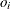is the offset for the ith observation, floor(exp()) should be greater than or equal to the response value. See the section Exact Logistic and Exact Poisson Regression for information about the use of the offset in the exact Poisson model.

PREDICTED
PRED
P

requests that predicted values, the linear predictor, its standard error, and the Hessian weight be displayed (see the OBSTATS option).

RESIDUALS
R

requests that residuals and standardized residuals be displayed. Residuals and other diagnostic statistics are not available for the multinomial distribution (see the OBSTATS option).

SCALE=number
SCALE=PEARSON
SCALE=P
PSCALE
SCALE=DEVIANCE
SCALE=D
DSCALE

sets the value used for the scale parameter where the NOSCALE option is used. For the binomial and Poisson distributions, which have no free scale parameter, this can be used to specify an overdispersed model. In this case, the parameter covariance matrix and the likelihood function are adjusted by the scale parameter. See the section Dispersion Parameter and the section Overdispersion for more information. If the NOSCALE option is not specified, then number is used as an initial estimate of the scale parameter.

Specifying SCALE=PEARSON or SCALE=P is the same as specifying the PSCALE option. This fixes the scale parameter at the value 1 in the estimation procedure. After the parameter estimates are determined, the exponential family dispersion parameter is assumed to be given by Pearson’s chi-square statistic divided by the degrees of freedom, and all statistics such as standard errors and likelihood ratio statistics are adjusted appropriately.

Specifying SCALE=DEVIANCE or SCALE=D is the same as specifying the DSCALE option. This fixes the scale parameter at a value of 1 in the estimation procedure.

After the parameter estimates are determined, the exponential family dispersion parameter is assumed to be given by the deviance divided by the degrees of freedom. All statistics such as standard errors and likelihood ratio statistics are adjusted appropriately.

SCORING=number

requests that on iterations up to number, the Hessian matrix be computed using the Fisher scoring method. For further iterations, the full Hessian matrix is computed. The default value is 1. A value of 0 causes all iterations to use the full Hessian matrix, and a value greater than or equal to the value of the MAXITER option causes all iterations to use Fisher scoring. The value of the SCORING= option must be 0 or a positive integer.

SINGULAR=number

sets the tolerance for testing singularity of the information matrix and the crossproducts matrix. Roughly, the test requires that a pivot be at least this number times the original diagonal value. By default, number is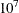times the machine epsilon. The default number is approximately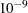on most machines. This value also controls the check on estimability for ESTIMATE and CONTRAST statements.

TYPE1

requests that a Type 1, or sequential, analysis be performed. This consists of sequentially fitting models, beginning with the null (intercept term only) model and continuing up to the model specified in the MODEL statement. The likelihood ratio statistic between each successive pair of models is computed and displayed in a table.

A Type 1 analysis is not available for GEE models, since there is no associated likelihood.

TYPE3

requests that statistics for Type 3 contrasts be computed for each effect specified in the MODEL statement. The default analysis is to compute likelihood ratio statistics for the contrasts or score statistics for GEEs. Wald statistics are computed if the WALD option is also specified.

WALD

requests Wald statistics for Type 3 contrasts. You must also specify the TYPE3 option in order to compute Type 3 Wald statistics.

WALDCI

requests that two-sided Wald confidence intervals for all model parameters be computed based on the asymptotic normality of the parameter estimators. This computation is not as time-consuming as the LRCI method, since it does not involve an iterative procedure. However, it is thought to be less accurate, especially for small sample sizes. The confidence coefficient can be selected with the ALPHA= option in the same way as for the LRCI option.

XVARS

requests that the regression variables be included in the OBSTATS table.